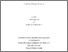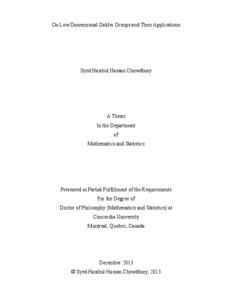Title:

# On Low Dimensional Galilei Groups and Their Applications

Chowdhury, Syed Hasibul Hassan (2013) On Low Dimensional Galilei Groups and Their Applications. PhD thesis, Concordia University.Preview Text (application/pdf) Chowdhury_PhD_S2014.pdf - Accepted Version 1MB

## Abstract

This thesis consists of two main parts. The first part focuses on the (1+1) and (2+1) dimensional Galilei groups and their applications to signal analysis and noncommutative quantum mechanics. Various groups used in the current literature of signal analysis and image processing turn out to possess deep connections with the (1+1)-dimensional Galilei group, which, on the other hand, is the physical kinematical symmetry group of a non-relativistic system in one spatial and one time dimensions. To study this remarkable representation theoretic similarity of structures is one of the many goals of this thesis. The (1+1)-affine Galilei group, a 2-fold noncentral extension of the Galilei group, is precisely responsible for the above-mentioned bridging. Wigner functions associated with another extension of (1+1) affine Galilei group are computed and their support properties are subsequently discussed along with a comparative study of those related to various centrally extended (1+1)-Galilei groups. The remainder of the first part of the thesis is devoted to the study of the (2+1)-Galilei group and its relationship to non-commutative quantum mechanics (NCQM). We show that a certain triple central extension of the abelian group of translations in $\mathbb{R}^{4}$ can be considered to be the {\em defining group} of NCQM in the same spirit as the Weyl-Heisenberg group is considered for the case of standard 2-dimensional quantum mechanics. The representations associated with various gauges studied in NCQM along with those of standard QM are all found to be sitting inside the unitary dual of the \textit{triply extended group of translations in $\mathbb{R}^{4}$}.\\
The second part of the thesis, which concerns an entirely separate problem, involves a study of Poisson brackets between traces of monodromy matrices computed along free homotopy classes of loops on a compact Riemann surface $\Sigma$. We consider a 3-manifold $\Sigma\times\mathbb{R}$ with the connection 1-forms taking their values in the Lie algebra $\mathcal{G}$ associated to the structure Lie group $G$ of a principal $G$-bundle defined on the base manifold $\Sigma\times\mathbb{R}$. First we apply the Hamiltonian formulation of the Chern-Simons theory to compute the Atiyah-Bott brackets between the relevant $\mathcal{G}$-valued relevant gauge connections. The quotient of this infinite dimensional space of flat connections by the action of gauge transformations is what one calls the {\em moduli space of flat connections}. Traces of monodromies computed along the free homotopy classes of loops on $\Sigma$ are the underlying gauge invariant observables. We compute Poisson brackets between observables of this sort by applying the Hamiltonian formalism of soliton theory for various real structure Lie groups, e.g. $GL(n,\mathbb{R})$, $SL(n,\mathbb{R})$, $U(n)$, $SU(n)$ and $Sp(2n,\mathbb{R})$. The formulae, thus obtained, are found to be in exact agreement with the ones computed by Goldman in \cite{goldmant}.

Divisions: Concordia University > Faculty of Arts and Science > Mathematics and Statistics Thesis (PhD) Chowdhury, Syed Hasibul Hassan Concordia University Ph. D. Mathematics December 2013 Ali, Syed Twareque 978056 SYED CHOWDHURY 16 Jun 2014 14:07 18 Jan 2018 17:45
All items in Spectrum are protected by copyright, with all rights reserved. The use of items is governed by Spectrum's terms of access.

Repository Staff Only: item control pageResearch related to the current document (at the CORE website)
Back to top